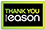# Applied numerical methods for chemical engineers

PAPERBACK
Applied Numerical Methods for Chemical Engineers emphasizes the derivation of a variety of numerical methods and their application to the solution of engineering problems, with special attention to problems in the chemical engineering field. These algorithms encompass linear and nonlinear algebraic equations, eigenvalue problems, finite difference methods, interpolation, differentiation and integration, ordinary differential equations, boundary value problems, partial differential equations, and linear and nonlinear regression analysis. MATLAB is adopted as the calculation environment throughout the book because of its ability to perform all the calculations in matrix form, its large library of built-in functions, its strong structural language, and its rich graphical visualization tools. Through this book, students and other users will learn about the basic features, advantages and disadvantages of various numerical methods, learn and practice many useful m-files developed for different numerical methods in addition to the MATLAB built-in solvers, develop and set up mathematical models for problems commonly encountered in chemical engineering, and solve chemical engineering related problems through examples and after-chapter problems with MATLAB by creating application m-files.
€100.73302 Reward Points
In stock online
Delivery in 2-3 working days

Any purchases for more than €10 are eligible for free delivery anywhere in the UK or Ireland!

Applied Numerical Methods for Chemical Engineers emphasizes the derivation of a variety of numerical methods and their application to the solution of engineering problems, with special attention to problems in the chemical engineering field. These algorithms encompass linear and nonlinear algebraic equations, eigenvalue problems, finite difference methods, interpolation, differentiation and integration, ordinary differential equations, boundary value problems, partial differential equations, and linear and nonlinear regression analysis. MATLAB is adopted as the calculation environment throughout the book because of its ability to perform all the calculations in matrix form, its large library of built-in functions, its strong structural language, and its rich graphical visualization tools. Through this book, students and other users will learn about the basic features, advantages and disadvantages of various numerical methods, learn and practice many useful m-files developed for different numerical methods in addition to the MATLAB built-in solvers, develop and set up mathematical models for problems commonly encountered in chemical engineering, and solve chemical engineering related problems through examples and after-chapter problems with MATLAB by creating application m-files.
Quantity:
In stock online
Delivery in 2-3 working days302 Reward Points

Any purchases for more than €10 are eligible for free delivery anywhere in the UK or Ireland!

€100.73
In stock online
Delivery in 2-3 working days